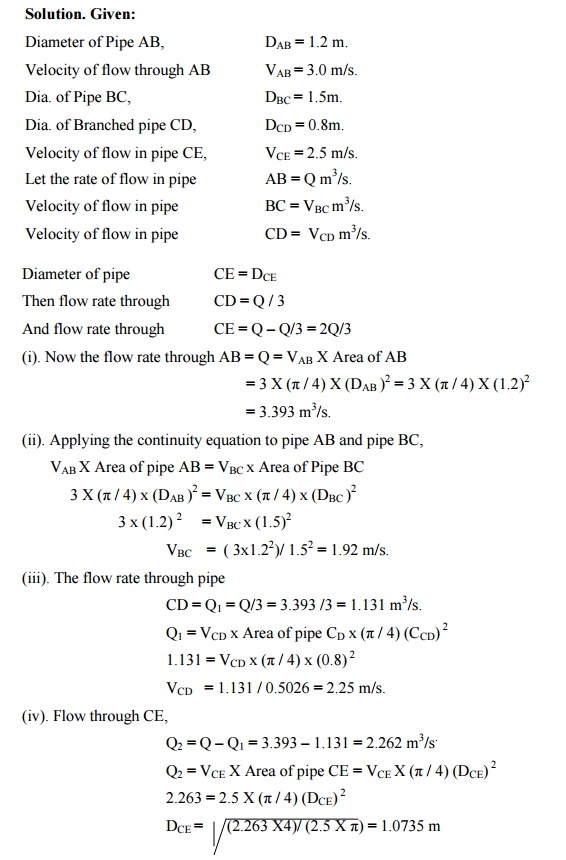Home | | Mechanics of Fluids | Darcy-Weibach Equation

# Darcy-Weibach Equation

Expression for loss of head due to friction in pipes or Darcy -Weisbach Equation.

Darcy-Weibach Equation

Expression for loss of head due to friction in pipes or Darcy -Weisbach Equation.

Consider a uniform horizontal pipe, having steady flow as shown figure. Let 1 -1 and 2-2 is two sections of pipe.

Let P1 = pressure intensity at section 1-1. Let P2 = Velocity of flow at section 1-1.

L = length of the pipe between the section 1-1 and 2-2 d = diameter off pipe.

f1 = Frictional resistance per unit wetted area per unit velocity. hf = loss of head due to friction.

And P2,V2 = are the values of pressure intensity and velocity at section 2-2.

Applying   Bernoulli's - 1 equation &2-2   between   section 1.1,1.2(previous)

Total head 1-1 = total head at 2-2 + loss of head due to friction between 1-1&2-2 (P1/?g) 1 2+/2g)(V+Z1 = (P2/?g)2 2 / +2g) +(VZ2+hf ------------(1)

but Z1 = Z1 [ pipe is horizontal ]

V1= V2 [ diameter of pipe is same at 1-1 & 2-2]

(1) becomes,

(P1/ ?g)2/?g)+h=f(P hf = (P1/ ?g)-(P2/?g)

frictional resistance = frictional resistance per unit wetted area per unit velocity X wetted area X velocity 2.

F = f1 x ?d l x V2 [ Wetted area = ?d x L, and Velocity V = V1 = V2] F1 = f1xPxLxV2 ----------- (2). [?d = wetted perimeter = p]

The forces acting on the fluid between section 1-1 and 2-2 are,

1) Pressure force at section 1-1 = P1X A

2) Pressure force at section 2-2 = P2 X A

3). Frictional force F1

Resolving all forces in the horizontal direction.,

P1 A -P2A -F1 = 0

(P1-P2)A = F1 = f1xPxLxV2

(P1-P2) = (f1xPxLxV2 / A ).

But from (1) we get

P1 -P2 = ?ghhf

Equating the values of (P1 -P2) we get

?ghf   = h(f1xPxLxV2 / A ).

hf = (f1  /   ?g)   X   (P/A)   X   LX   V2

(P/A) = (?d / (?d2/4)) = (4/d)

Hence, hf = ( f1 /   ?g)   x   (.   4/d)   x   LxV2

hf = 4 fLV 2 / 2gd

This equation is known as Darcy -Weisbach equation. This equation is commonly used to find loss of head due to friction in pipes.

Problem

Water flows through a pipe AB 1.2m diameter at 3 m/s and then passes through a pipe BC 1.5 m diameter at C, the pipe branches. Branch CD is 0.8m in diameter and carries one third of the flow in AB. The flow velocity in branch CE is 2.5 m/s. Find the volume rate of flow in AB, the velocity in BC, the velocity in CD and the diameter of CE.Study Material, Lecturing Notes, Assignment, Reference, Wiki description explanation, brief detail
Civil : Mechanics Of Fluids : Flow Through Pipes : Darcy-Weibach Equation |